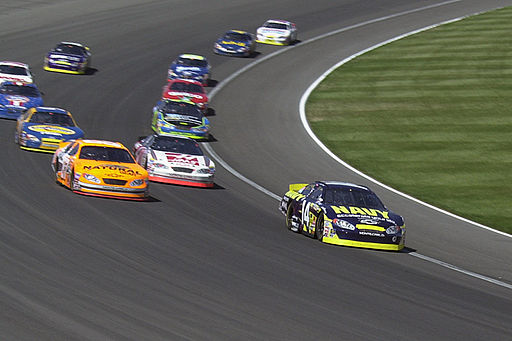# Difference Between Velocity and Acceleration

## Main Difference – Velocity vs. Acceleration

Velocity and acceleration are both vector quantities related to the motion of an object. The main difference between velocity and acceleration is that velocity is the rate of change of displacement while acceleration is the rate of change of velocity.

## What is Velocity

If the position of a body can be represented by a position vector$\vec{r}$, then the velocity$\vec{v}$ of the body is defined as:$\vec{v}=\frac{\mathrm{d}\vec{r}}{\mathrm{d}t}$

This gives the instantaneous velocity of an object, i.e. the velocity at a specific time. The average velocity is given by:$\mathrm{average\:velocity=\frac{displacement}{time\:taken}}$

Speed is the magnitude of an object’s velocity. The average speed is the total distance travelled by the body divided by the time taken. If an object travels some distance and comes back to its starting point, the body would have an average speed but its average velocity will be 0 since its displacement is 0. Since velocity is a vector quantity, a change in velocity involves either a change in an object’s speed or a change in its direction of motion.These cars are accelerating since they are changing their direction of motion even though their speed may remain the same.

The SI unit for measuring velocity is m s-1.

## What is Acceleration

Acceleration is defined as the rate of change of velocity:$\vec{a}=\frac{\mathrm{d}\vec{v}}{\mathrm{d}t}=\frac{\mathrm{d}^2\vec{r}}{\mathrm{d}t^2}$

According to Newton’s second law of motion, a resultant force is necessary to produce an acceleration. If the direction of acceleration is in the opposite direction to the body’s direction of motion, the body is under deceleration and its speed decreases.

The SI unit for measuring acceleration is m s-2.

Bodies falling under gravity close to Earth’s surface are approximately falling at a constant acceleration of about 9.81 m s-2. This acceleration is given the symbol$g$. Sometimes,$g$ is used as a unit to express acceleration. If a human is exposed to an acceleration higher than about$5g$, blood may not flow into the brain the person might lose consciousness. This can happen, for example, in roller-coasters achieving high accelerations. Astronauts and aviators need to be trained to stay conscious at high-$g$ accelerations. The video below shows a trainee losing consciousness from an acceleration of$9g$ (Note: GLOC stands for “$g$-induced Loss Of Consciousness”).

## Difference Between Velocity and Acceleration

### Definition

Velocity is the rate of change of displacement:$\vec{v}=\frac{\mathrm{d}\vec{r}}{\mathrm{d}t}$.

Acceleration is the rate of change of velocity:$\vec{a}=\frac{\mathrm{d}\vec{v}}{\mathrm{d}t}=\frac{\mathrm{d}^2\vec{r}}{\mathrm{d}t^2}$.

### Units

Velocity has units m s-1.

Acceleration has units m s-2.

Image Courtesy:

“ Fontana, Calif. (May 1, 2004) – The U.S. Navy sponsored Chevy Monte Carlo NASCAR…” by Chief Journalist Erik Schneider. (U.S. Navy photo ID 040501-N-1336S-037) [Public domain], via Wikimedia Commons